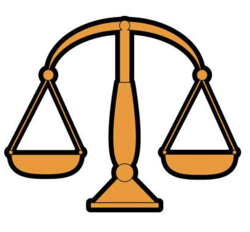#8 Balls Weight Puzzle (Solved)

This is my favorite weight puzzle which have been asked from me in many interviews over the past few years.

## Puzzle

You have 8 balls identical in size and appearance. One of them is defective and weighs heavy than the others. You have a weighing scale with no measurements so you can just compare weight of balls against each other. How would you find the defective ball given only 2 chances to use the scale?

## Solution

First of all we will give a number to each ball i.e. 1, 2, 3, 4, 5, 6, 7, and 8

The trick to solve these kind of weight problem is to divide them in groups. We will divide these 8 balls in 3 groups:

GroupBall Numbers
group 11, 2, 3
group 24, 5, 6
group 37, 8

Now we keep the group 3 aside and put group 1 balls on one side of scale and group 2 balls on another side of scale [1st chance] with three possible outcomes:-

#### 1. Scale is balanced

That means each ball in group 1 and group 2 are identical in weight and defective one is from group 3.

Let’s put group 3 balls 7 and 8 on each side of scale [2nd chance] with two possible outcomes:-

1. If 7 is heavy then it is defective one
2. If 8 is heavy then it is defective one

#### 2. group 1 is heavier then group 2

That means defective balls is from group 1 i.e. either 1 or 2 or 3.

Let’s keep number 3 aside and put balls 1 and 2 on each side of scale [2nd chance] with three possible outcomes:-

1. If 1 and 2 balances, then 3 is defective one
2. If 1 is heavy then it is defective one
3. If 2 is heavy then it is defective one

#### 3. group 2 is heavier then group 1

That means defective balls is from group 2 i.e. either 4 or 5 or 6.

Let’s keep number 6 aside and put balls 4 and 5 on each side of scale [2nd chance] with three possible outcomes:-

1. If 4 and 5 balances, then 6 is defective one
2. If 4 is heavy then it is defective one
3. If 5 is heavy then it is defective one

## Conclusion

That’s it champs. We have found the defective balls out of 8 balls in only 2 weighing.

If by now you understood the trick of dividing balls in group and keeping some balls aside then you can solve weight puzzle with any number of balls. Here is the cheat sheet:-

###### Cheat Sheet
N Balls Weight PuzzleGroupsMin Weighing (Best Case)Min Weighing (Worst Case)
N = 2 11
N = 3  11
N = 4  [3,4]12
N = 5[1,2] [3,4] 12
N = 6[1,2] [3,4] [5,6]22
N = 6[1,2,3] [4,5,6] 12
N = 8[1,2,3] [4,5,6] [7,8]22
N = 9[1,2,3] [4,5,6] [7,8,9]22
N = 10[1,2,3,4] [5,6,7,8] [9,10]23
N = 11[1,2,3,4] [5,6,7,8] [9,10,11]23
N = 12[1,2,3,4] [5,6,7,8] [9,10,11,12]33

If you are interested in how to solve 9 or 12 balls weight puzzle then check out these posts:-